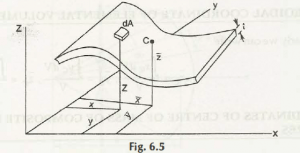Fig. 6.5 describes an area of constant thickness which is denoted by t. Therefore the mass of elemental i.e. dm is given by

dm = ptdA                  p, t are constants, dA =elemental area.From equation (6.2), we have

x = ʃ x dA / A , y = ʃ y dA / A , z = ʃ z dA / A

In equation (6.6), ʃ x dA, ʃ y dA, ʃ z dA are referred as first moment of area.

Links of Next Mechanical Engineering Topics:-### Customer Reviews

My Homework Help
Rated 5.0 out of 5 based on 510 customer reviews at